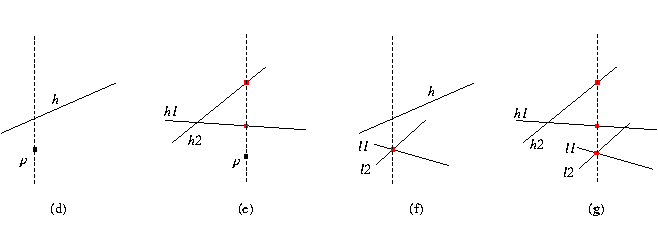## CGAL::compare_y_at_x

Depending on which CGAL kernel is used, different versions of this global function are available. This is described below.

With the basic 2D and 3D Kernel (see Chapter 7)

Comparison_result compare_y_at_x ( Point_2<Kernel> p, Line_2<Kernel> h)
compares the y-coordinates of p and the vertical projection of p on h, see (d) in the figure below.
 Precondition: h is not vertical.

Comparison_result compare_y_at_x ( Point_2<Kernel> p, Line_2<Kernel> h1, Line_2<Kernel> h2)
compares the y-coordinates of the vertical projection of p on h1 and on h2, see (e) in the figure below.
 Precondition: h1 and h2 are not vertical.

Comparison_result compare_y_at_x ( Line_2<Kernel> l1, Line_2<Kernel> l2, Line_2<Kernel> h)
Let p be the intersection of lines l1 and l2. This function compares the y-coordinates of p and the vertical projection of p on h, see (f) in the figure below.
 Precondition: l1, l2 intersect and h is not vertical.

Comparison_result compare_y_at_x ( Line_2<Kernel> l1, Line_2<Kernel> l2, Line_2<Kernel> h1, Line_2<Kernel> h2)
Let p be the intersection of lines l1 and l2. This function compares the y-coordinates of the vertical projection of p on h1 and on h2, see (g) in the figure below.
 Precondition: l1 and l2 intersect; h1 and h2 are not vertical.

Comparison_result compare_y_at_x ( Point_2<Kernel> p, Segment_2<Kernel> s)
compares the y-coordinates of p and the vertical projection of p on s. If s is vertical, then return EQUAL when p lies on s, SMALLER when p lies under s, and LARGER otherwise.
 Precondition: p is within the x range of s.

Comparison_result compare_y_at_x ( Point_2<Kernel> p, Segment_2<Kernel> s1, Segment_2<Kernel> s2)
compares the y-coordinates of the vertical projection of p on s1 and on s2. If s1 or s2 is vertical, then return EQUAL if they intersect, otherwise return SMALLER if s1 lies below s2, and return LARGER otherwise.
 Precondition: p is within the x range of s1 and s2.With the 2D Circular Kernel (see Chapter 9)

#include <CGAL/global_functions_circular_kernel_2.h>

If this kernel is used, in addition to the function and the combination of 2D types described above, another version of the function is provided.

 Comparison_result compare_y_at_x ( Circular_arc_point_2 p, Circular_arc_2 a) Same as above, for a point and a circular arc. Comparison_result compare_y_at_x ( Circular_arc_point_2 p, Line_arc_2 a) Same as above, for a point and a line segment.